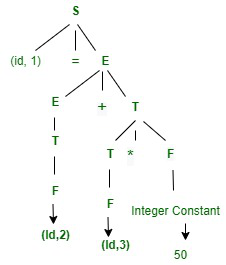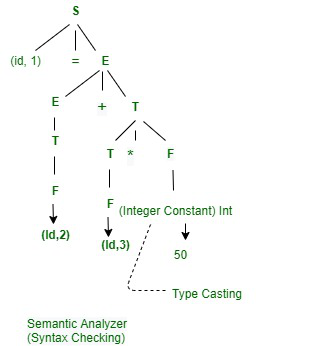# Working of Compiler Phases with Example

• Difficulty Level : Basic
• Last Updated : 24 Aug, 2020

In this article, we are going to cover an overview that how we can each compiler phase works individually with the help of an example. Let’s discuss one by one.

Pre-requisite – Introduction to compiler phases

You will see how compiler phases like lexical analyzer, Syntax analyzer, Semantic Analyzer, Intermediate code generator, code Optimizer, and Target code generation. let’s consider an example.

`x = a+b*50`

The symbol table for the above example is given below. In symbol table are clearly mentions the variable name and variable types.

S.No. Variable name Variable type
1 x float
2 a float
3 b float

Now, here you will see how you can execute the compiler phase at each level and how it works.

1. Lexical Analyzer :

In this phase, you will see how you can tokenize the expression.

```x  ->  Identifier-  (id, 1)
=  ->  Operator  -  Assignment
a  ->  Identifier-  (id, 2)
+  ->  Operator  -  Binary Addition
b  ->  Identifier-  (id, 3)
*  ->  Operator  -  Multiplication
50 ->  Constant  -  Integer```

Now, the final tokenized expression is given below.

`(id, 1) = (id, 2) + (Id, 3)*50`
2. Syntax Analyzer :

In this phase, you will see how you can check the syntax after tokenized the expression.

```S -> Id = E
E -> E+T | T
T -> T*F | F
F -> Id | Integer constant```

SDT for the above expression is given below.3. Semantic Analyzer :
In this phase, you will see how you can check the type and semantic action for the syntax tree. Given below is the diagram of the semantic analyzer.4. Intermediate Code Generator :
In this phase as an input, you will give a modified parse tree and as output after converting into Intermediate code will generate 3 -Address Code. Given below is an expression of the above-modified parse tree.

```t1 = b * 50.0
t2 = a+t1
x = t2```
5. Code Optimizer :
In this phase, you will see as an input will give 3 address code and as an output, you will see optimize code. Let’s see how it will be converted.

```t1 = b* 50.0
x = a+ t1```
6. Target Code Generator :
It is the last phase and In this, you will see how you can convert the final expression into assembly code. so, that it will be easy to understand for the processor.

```Mul